# Gauge Transformations

## Homework Statement

For a gauge function G(t,q) where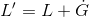,
does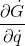or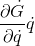have any alternative form or can they be expressed in any other way?

## The Attempt at a Solution

stevendaryl
Staff Emeritus
Could you give a little more context to your question? I assume you're talking about electromagnetism? Or are you talking about quantum field theory? What is $L$ and $L'$?

Its a mechanical system, but just working on proofs and theory so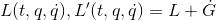I'm working on proving how different quantities change, such as the euler lagrange equations, the generalised momenta, mechanical energy and power

So for example for the euler lagrange I have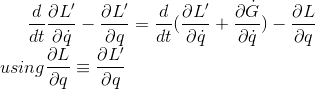correction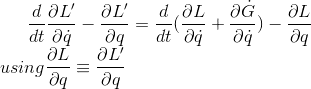stevendaryl
Staff Emeritus
Oh, so you're just talking about Lagrangian mechanics.

If $G$ is a function of $q$ and $t$, then you have:

$\dot{G} = \frac{\partial G}{\partial q} \dot{q} + \frac{\partial G}{\partial t}$

So in that case, $\frac{\partial \dot{G}}{\partial \dot{q}} = \frac{\partial G}{\partial q}$

Oh wow, this is painful how much I overlooked that. Thanks!

Oh, so you're just talking about Lagrangian mechanics.

If $G$ is a function of $q$ and $t$, then you have:

$\dot{G} = \frac{\partial G}{\partial q} \dot{q} + \frac{\partial G}{\partial t}$

So in that case, $\frac{\partial \dot{G}}{\partial \dot{q}} = \frac{\partial G}{\partial q}$

A quick question about the same function, would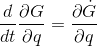be a true statement?

stevendaryl
Staff Emeritus
A quick question about the same function, wouldbe a true statement?

Yes, if $G$ is only a function of $q$ and $t$.

Yes, if $G$ is only a function of $q$ and $t$.

G is a function of q and t, (G(t,q) to be exact). Could you explain why you can change the order of the derivatives in this case?

stevendaryl
Staff Emeritus
If you have a function $X(t,q)$of $q$ and $t$, then $\dot{X} = \frac{d}{dt} X = (\frac{\partial}{\partial t} + \frac{\partial}{\partial q} \frac{dq}{dt}) X = (\frac{\partial}{\partial t} + \frac{\partial}{\partial q} \dot{q}) X$
So $\dot{G} = \frac{\partial G}{\partial t} + \dot{q} \frac{\partial G}{\partial q}$. Therefore, $\frac{\partial \dot{G}}{\partial q} = \frac{\partial^2 G}{\partial t \partial q} + \dot{q} \frac{\partial^2 G}{\partial q^2}$.
Similarly, $\frac{d}{dt} \frac{\partial G}{\partial q} = (\frac{\partial}{\partial t} + \frac{\partial}{\partial q} \dot{q}) \frac{\partial G}{\partial q} = \frac{\partial^2 G}{\partial t \partial q} + \frac{\partial^2 G}{\partial q^2} \dot{q}$
•